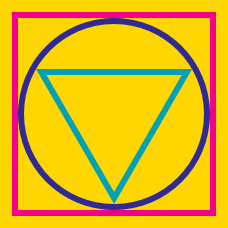Geometry

# Circumcircle of Triangle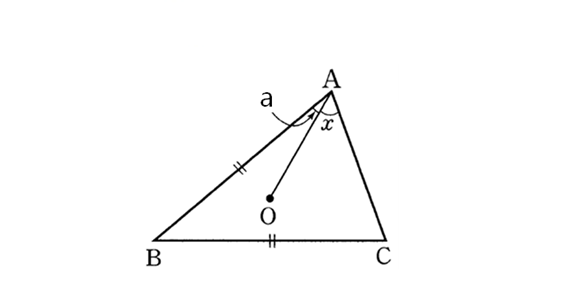In the above diagram, point $O$ is the circumcenter of $\triangle ABC$ and the lengths of $\overline{AB}$ and $\overline{BC}$ are the same, i.e. $\lvert \overline{AB} \rvert=\lvert \overline{BC} \rvert.$ If $\angle OAB(=a)$ is $15^{\circ},$ what is $\angle OAC(=x)$?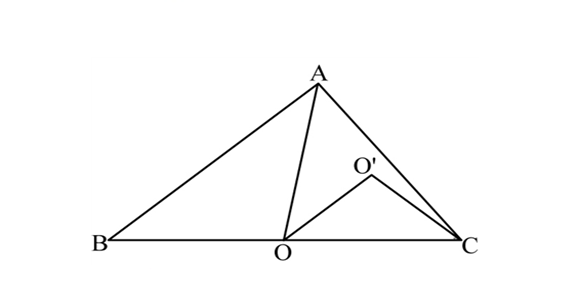In the above diagram, point $O$ is the circumcenter of $\triangle ABC$ and point $O'$ is the circumcenter of $\triangle AOC.$ If $\angle ABO = 28^{\circ},$ what is the value of $\angle OO'C$ in degrees?

Note: The above diagram is not drawn to scale.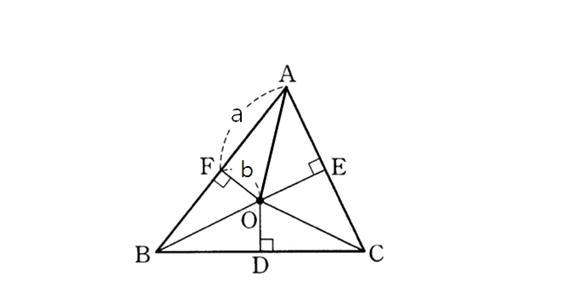In the above diagram, point $O$ is the circumcenter of $\triangle ABC.$ The length of $\overline{AF}$ is $10(=a)$ and the length of $\overline{OF}$ is $3(=b).$ If the area of $\triangle ABC$ is $116,$ what is the area of $CDOE?$

Note: The above diagram is not drawn to scale.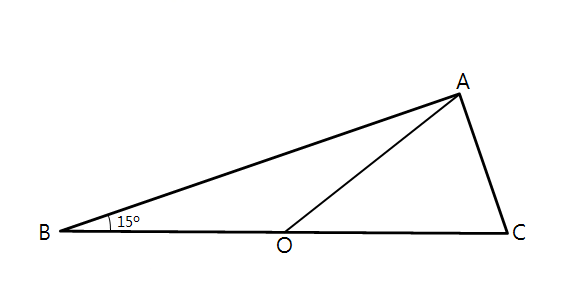In the above diagram, point $O$ is the circumcenter of $\triangle ABC$ and $\angle ABC$ is $15^{\circ}.$ If the radius of the circumscribed circle is $16,$ what is the area of $\triangle ABC?$

Note: The above diagram is not drawn to scale.In the above diagram, point $O$ is the circumcenter of $\triangle ABC.$ The length of $\overline{AF}$ is $6(=a)$ and the length of $\overline{OF}$ is $5(=b).$ If the area of $\triangle ABC$ is $98,$ what is the area of $CDOE?$

Note: The above diagram is not drawn to scale.

×